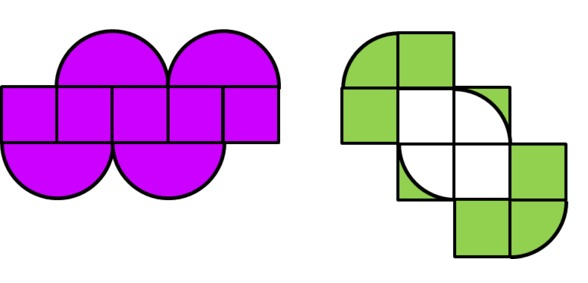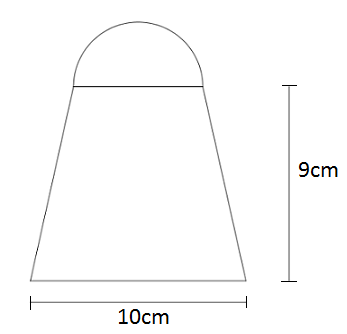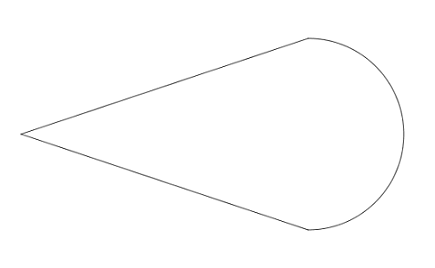# Geometry

## Objective

Solve problems involving area and circumference of two-dimensional figures (Part 1).

## Common Core Standards

### Core Standards

?

• 7.G.B.4 — Know the formulas for the area and circumference of a circle and use them to solve problems; give an informal derivation of the relationship between the circumference and area of a circle.

• 7.G.B.6 — Solve real-world and mathematical problems involving area, volume and surface area of two- and three-dimensional objects composed of triangles, quadrilaterals, polygons, cubes, and right prisms.

?

• 6.G.A.1

## Criteria for Success

?

1. Know and use the formulas for area and circumference of a circle.
2. Given the circumference of a circle, determine the area of the circle.
3. Find the area and perimeter/circumference of composite shapes, including shapes composed of circles, semi-circles, quadrilaterals, and triangles.

## Tips for Teachers

?

• Lessons 10 and 11 engage students in finding area and circumference/perimeter measurements of different geometric shapes including circles, quadrilaterals, and triangles. The Problem Set Guidance includes a variety of problems that range in difficulty and time required.
• Students engage in MP.1 and MP.7 as they make sense of complex geometric figures, looking for significance of shapes and measurements within the structure of the diagram, and looking for entry points to solve the problem. Support students by asking questions about what they observe in the diagrams and what initial strategy ideas they have.

#### Remote Learning Guidance

If you need to adapt or shorten this lesson for remote learning, we suggest prioritizing Anchor Problem 3 (benefits from worked example). Find more guidance on adapting our math curriculum for remote learning here.

#### Fishtank Plus

• Problem Set
• Student Handout Editor
• Vocabulary Package

## Anchor Problems

?

### Problem 1

The circumference of a circle is $24\pi$ cm. What is the exact area of the circle? Draw a diagram to assist you in solving the problem.

#### References

EngageNY Mathematics Grade 7 Mathematics > Module 3 > Topic C > Lesson 18Example 3

Grade 7 Mathematics > Module 3 > Topic C > Lesson 18 of the New York State Common Core Mathematics Curriculum from EngageNY and Great Minds. © 2015 Great Minds. Licensed by EngageNY of the New York State Education Department under the CC BY-NC-SA 3.0 US license. Accessed Dec. 2, 2016, 5:15 p.m..

### Problem 2

Find the area and perimeter of the colored part of each figure below. The figures are composed of small squares with side length 1 unit and curves that are an arc of a circle.#### References

Illustrative Mathematics Designs

Designs, accessed on March 10, 2018, 12:59 p.m., is licensed by Illustrative Mathematics under either the CC BY 4.0 or CC BY-NC-SA 4.0. For further information, contact Illustrative Mathematics.

Modified by The Match Foundation, Inc.

### Problem 3

A semi-circle with a diameter of 6 cm sits on top of an isosceles trapezoid. Find the area of the entire figure.## Problem Set

?The following resources include problems and activities aligned to the objective of the lesson that can be used to create your own problem set.